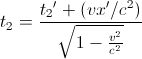## Pages

### S10. Time Dilation

We now move onto the topic of Time Dilation. We can imagine the situation below to illustrate the phenomenon of the time dilation and come up with the quantitative description of the time dilation.

Imagine a gun placed at the position $({x}', {y}',{z}',{t}')$ in ${S}'$. Suppose it fires two shots at time  ${t_{1}}'$ and ${t_{2}}'$ measured with respect to ${S}'$. In ${S}'$ the clock is at rest relative to the observer. The time interval measured by a clock at rest relative to the observer is called the proper time interval.

Hence, ${t_{0}}={{t_{2}}'}-{{t_{1}}'}$ is the time interval between the two shots for the observer in ${S}'$. Since the gun is fixed in ${S}'$, it has the velocity $v$ with respect to ${S}$ in the direction of the positive ${X}$-axes.

Let, ${t}={{t_{2}}}-{{t_{1}}}$ represent the time interval between the two shot as measured by an observer in ${S}$.

We, know from the Lorentz Transformation the following transformation for the time coordinate,$(2)$

Note: We have made use of the reverse lorentz Transformation. Now, Subtracting the two equation we have the following,

Then,

Replacing the measured time interval in the ${S}$ and ${S}'$ as the $t$ and $t_{0}$, we have the final form of the equation,

We have thus found an expression for the time dilation.

One of the well know experiment of muon decay clearly illustrate us the validity of the concept of the length contraction and the time dilation phenomenon. Other very well know and interesting experiments is the Hafele and Keating time dilation experiment. Details can be read here and on the wikipedia.

You can find further note from the following sites,